### 3.5. Mean Curvature Blur

#### 3.5.1. Overview

Regularize geometry at a speed proportional to the local mean curvature value: this filter uses the mean curvature algorithm to blur the image preserving edges.

The Mean Curvature algorithm is described on Wikipedia.

#### 3.5.2. Activate the filter

You can find this filter in the image menu under FiltersBlurMean Curvature Blur…

#### 3.5.3. Options

Figure 17.16. Mean Curvature Blur filter parameters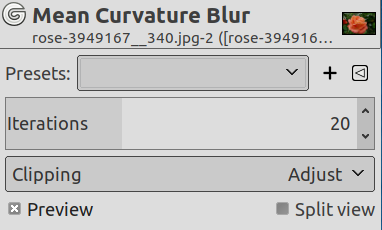Presets, Input Type, Clipping, Blending Options, Preview, Split view
NoteThese options are described in Section 2, “Common Features”.
Iterations

Increasing Iterations increases blur.

#### 3.5.4. Examples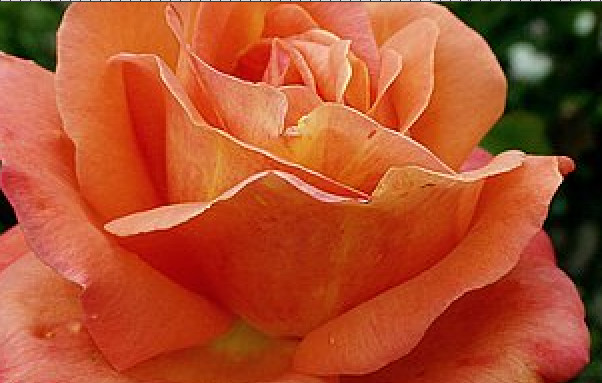Original image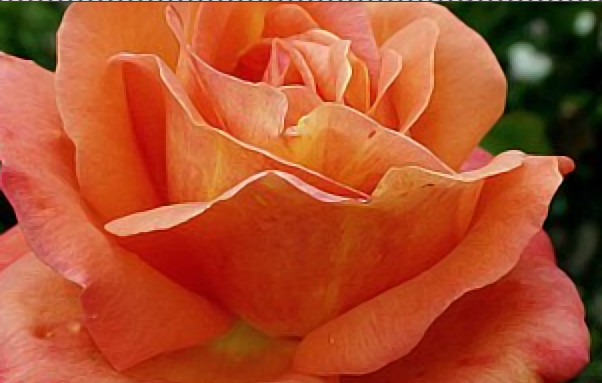Iterations = 4

Mean Curvature Blur can be used to denoise an image:

Figure 17.17. Mean Curvature Blur to denoise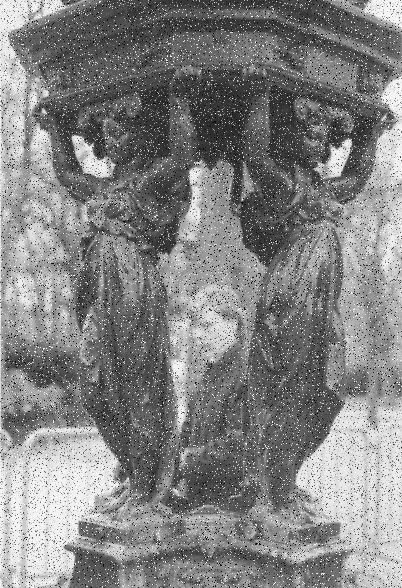Original image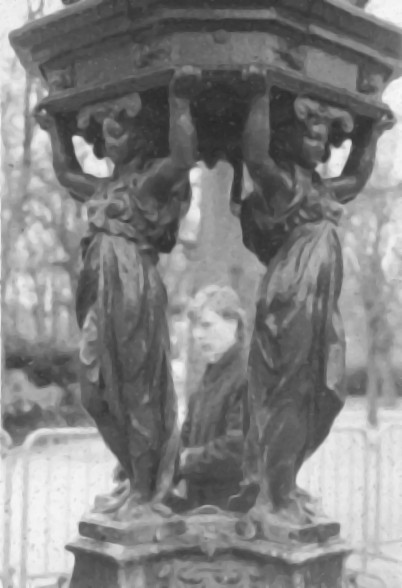Iterations = 9